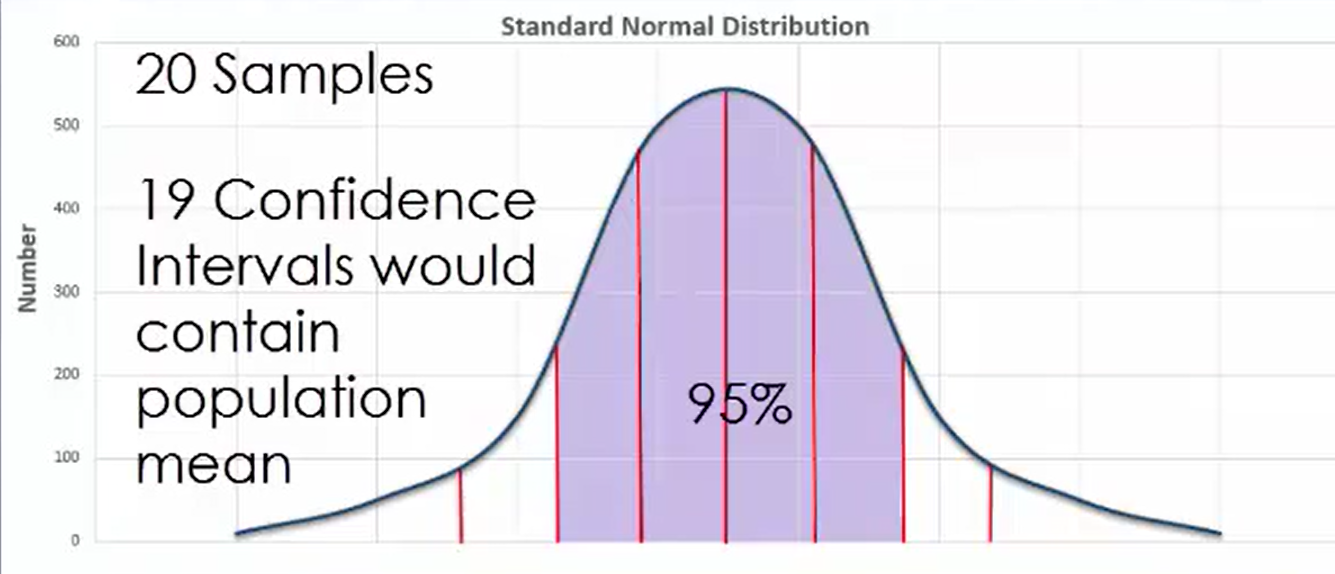+
Applying Confidence Intervals
Interpret Inferential Statistics
0
of 0 possible points

# Applying Confidence Intervals

Author: Sophia Tutorial
##### Description:

Explain the 95% confidence interval in a given scenario.

(more)

Sophia’s self-paced online courses are a great way to save time and money as you earn credits eligible for transfer to many different colleges and universities.*

No credit card required

37 Sophia partners guarantee credit transfer.

299 Institutions have accepted or given pre-approval for credit transfer.

* The American Council on Education's College Credit Recommendation Service (ACE Credit®) has evaluated and recommended college credit for 32 of Sophia’s online courses. Many different colleges and universities consider ACE CREDIT recommendations in determining the applicability to their course and degree programs.

Tutorial
what's covered
This lesson discusses applying confidence intervals. By the end of this lesson, you should be able to interpret a 95% confidence interval. This lesson covers:
1. Populations and Samples
2. Applying Confidence Intervals

## 1. Populations and Samples

Recall from previous lessons that a population is a entire pool of observations, while a sample is a small group of observations drawn from a population. It is desirable to randomly select that sample so as to get a relatively representative portion of the population, which allows you to make more accurate predictions about the population as a whole.

The mean of a data set gives an idea of the center of the data, regardless of whether the data set is a population or a sample. Typically, the likely range for a population mean is the 95% confidence interval. This means that if enough random samples are drawn from the population, the actual population mean will occur in this range in approximately 95% of the samples. To put it another way, if you were to conduct 20 experiments, 19 of the confidence intervals you obtain would contain the actual population mean.It is important to note that the population mean is a fixed value that does not change, and that you are simply trying to estimate it by using random samples.

## 2. Applying Confidence Intervals

Suppose you were to take a sample of 100 adults and measure how tall they were in the attempt to estimate the mean height of all adults. For this scenario, the sample mean of the height of 100 of adults is 67.5 inches with a 95% confidence interval being approximately 59.1 inches to 75.9 inches.

Now suppose you drew another sample of 100 adults, and it had a sample mean of 68.3 inches with a 95% confidence interval of 59.9 inches to 76.7 inches. If you continued drawing random samples of 100 adults, you would most likely arrive at different sample means and 95% confidence intervals for each instance.

Maybe your third sample has a sample mean of 67.9 inches and a 95% confidence interval of 59.5 inches to 76.3 inches, and a fourth sample is drawn with a sample mean of 66.1 inches and a 95% confidence interval of 57.7 inches to 74.5 inches.

If you were to continue drawing samples to the point where you had 100 of them, what would you expect to find?

You would expect the actual population mean to fall in the range of 95% of those samples.

here’s another example. Suppose you were interested in determining the mean number of cups of coffee that Americans drink per day, and you drew multiple random samples of 250 people in an effort to estimate this mean. Let’s suppose that the sample mean is 2.1 cups per day with a 95% confidence interval of 1.8 cups to 2.4 cups per day.

A second sample of 250 people yields a sample mean of 1.8 cups per day and a 95% confidence interval of 1.5 cups to 2.1 cups per day. If you repeat this process to the point of drawing 60 samples, you would expect that the actual population mean would fall in the range of 95% of those samples, or 57 of them.

summary
Populations and samples do not always have the same means. The confidence interval is how you can express what the population mean might be. You also practiced applying the confidence interval to real world scenarios to understand this concept further.

Source: This work is adapted from Sophia author Dan Laub.

Rating